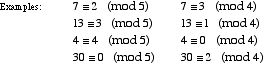# modular equivalence

Modular Equivalence

Two integers are equivalent mod n if they leave the same remainder when divided by n.

For example, 5 and 17 are equivalent mod 4 since they both have remainder 1 when divided by 4. We write
5 ≡ 17 (mod 4).